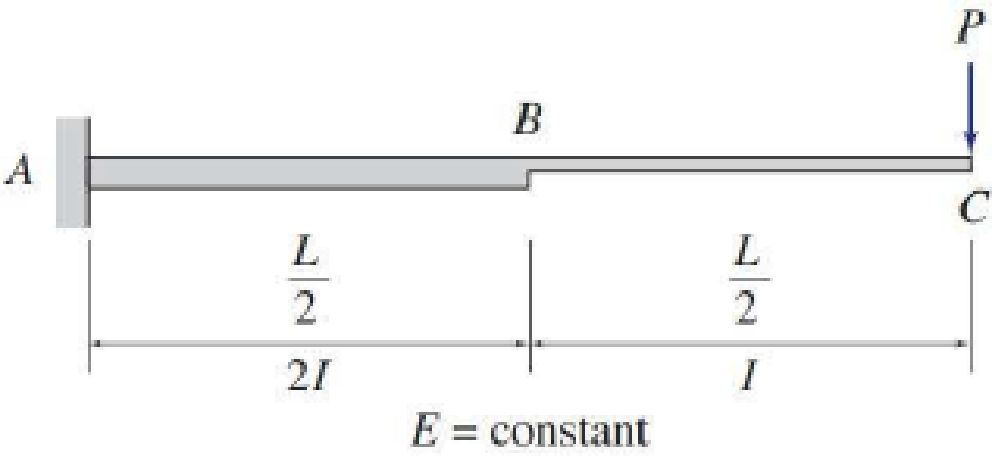# 6.40 through 6.43 Use the Conjugate-beam method to determine the slopes and deflections at points B and C of the beams Figs. P6.14 through P6.17. FIG. P6.14, P6.40

#### Solutions

Chapter
Section
Chapter 6, Problem 40P
Textbook Problem
682 views

## 6.40 through 6.43 Use the Conjugate-beam method to determine the slopes and deflections at points B and C of the beams Figs. P6.14 through P6.17.FIG. P6.14, P6.40To determine

Find the slope θB&θC and deflection ΔB&ΔC at point B and C of the given beam using the conjugate-beam method.

### Explanation of Solution

Calculation:

Consider modulus of elasticity E of the beam is constant.

Show the free body diagram of the given beam as in Figure (1).

Refer Figure (1),

Consider upward is positive and downward is negative.

Consider clockwise is negative and counterclockwise is positive.

Since the point C is free end there is no support reaction at Point C. Therefore, the reaction at point C is also equal to zero.

Determine the reaction at support A using the Equation of equilibrium;

V=0RAP=0RA=P

Determine the moment at point A;

MA(P×(L2+L2))=0MA=PL

Determine the moment at point B;

MB(P×(L2))=0MB=PL2

Show the M/EI diagram for the real beam as in Figure (2).

Conjugate-beam method:

In the given beam system, point A is a fixed end and point B is free end. But in the conjugate-beam method the fixed end of a real beam becomes free and the free end of real beam changed into the fixed end.

Show the M/EI diagram for the conjugate-beam as in Figure (3).

Calculation of shear at B in the conjugate-beam:

The shear force at B of the conjugate beam is equal to the slope at B on the real beam.

Consider the external forces acting (left of B) upward on the free body diagram as positive.

Determine the shear force at B using the relation;

SB=(bh)(12×b×h)

Here, b is the width and h is the height of the triangle and rectangle.

Substitute L2 for b and PL4EI and PL4EI for h.

SB=(L2×PL4EI)(12×L2×PL4EI)=PL28EIPL216EI=2PL2PL216EI=3PL216EI

Hence, the slope at point B is 3PL216EI(Clockwise)_.

The bending moment at B in the conjugate beam is equal to defection at B on the real beam.

Take the clockwise moments of the external forces about B as positive.

Determine the bending moment B using the relation;

MB=(bh)(b2)(12×b×h)(23×b)

Substitute L2 for b and PL4EI and PL4EI for h.

MB=(L2×PL4EI)(L/22)(12×L2×PL4EI)(23×L2)=PL332EIPL348EI=5PL396EI=5PL396EI()

Hence, the deflection at B is 5PL396EI()_

### Still sussing out bartleby?

Check out a sample textbook solution.

See a sample solution

#### The Solution to Your Study Problems

Bartleby provides explanations to thousands of textbook problems written by our experts, many with advanced degrees!

Get Started

Find more solutions based on key concepts
Draw the top, the front and the right side orthogonal views of the given object and indicate when an object nee...

Engineering Fundamentals: An Introduction to Engineering (MindTap Course List)

How is an incident response plan different from a disaster recovery plan?

Principles of Information Security (MindTap Course List)

Cloud Services Virtualization (IaaS) An online businesss website receives higher traffic during peak holiday sh...

Enhanced Discovering Computers 2017 (Shelly Cashman Series) (MindTap Course List)

Why must welding cables never be tied to scaffolding or ladders?

Welding: Principles and Applications (MindTap Course List)

If your motherboard supports ECC DDR3 memory, can you substitute non-ECC DDR3 memory?

A+ Guide to Hardware (Standalone Book) (MindTap Course List)